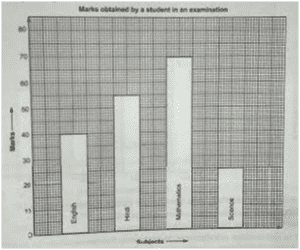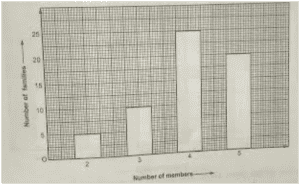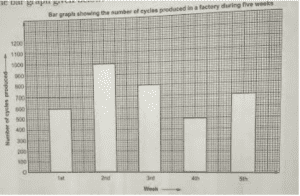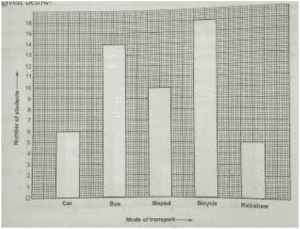RS Aggarwal Class 6 Solutions Chapter 24 Bar Graph

RS Aggarwal Class 6 Chapter 24 Bar Graph Solutions Free PDF

Bar Graph is one of the most important topics of mathematics. In class 6 the students will learn the basics of bar graph like interpretation of bar graph and drawing a bar graph. To understand the concepts of bar graphs and to learn the concepts in a better and easy way students are advised to solve questions from the RS Aggarwal for class 6. Students can also refer to RS Aggarwal class 6 solutions chapter 24 bar graph.

Exercise 24

QUESTION – 1: Look at the bar graph given below.(i) What information does the bar graph give?

(ii) In which subject is the student poorest?

(iii) In which subject is the student best?

(iv) In which subjects did he get more than 40 marks?

Solution:

(i) The given bar graph provides information about the marks obtained by a student in an examination in four different subjects.

(ii) The student is poorest in science because the height of the bar representing the marks obtained in science is the lowest.

(iii) The student is best in mathematics because the height of the bar representing the marks obtained in mathematics is the highest.

(iv) In Hindi and mathematics he got more than 40 marks. He scored 55 marks in Hindi and 70 marks in mathematics.

QUESTION – 2: In a survey of 60 families of a colony, the number of members in each family was recorded and the data has been represented by the bar graph given below:(i) What information does the bar graph give?

(ii) How many families have 3 members?

(iii) How many couples have no child?

(iv) Which type of family is the most common ?

Solution:

(i) The given graph provides information about the number of members in 60 families of a colony.

(ii) There are 10 families that have 3 members.

(iii) There are 5 couples that do not have children.

(iv) The families having two children are most common because of the bar of the families having four members has the highest height.

QUESTION – 3: Look at the bar graph given below:Study the bar graph carefully and answer the questions given below:

(i) In which week was the production maximum?

(ii) In which week was the production minimum?

(iii) What is the average production during these five weeks?

(iv0 How many cycles were produced in the first 3 weeks?

Solution:

(i) Production was maximum in the 2nd week.

(ii) Production was minimum in the 4th week.

(iii) Average production of these five weeks = Total production of all weeks / Number of weeks

= $frac{1000+600+800+500+700}{5}$

= 3600/5

= 720

(iv) Number of cycles produced in the first 3 week = Production in the 1st week + Production in the 2nd week + Production in the 3rd week

= 600 + 1000 + 800

= 2400 cycles

QUESTION – 4: 51 students from a locality use different modes of transport to school, as shown by the bar graph given below:Look at the bar graph given above and answer the questions given below:

(i) What does the above bar graph show?

(ii) Which mode of transport is used by maximum number of students?

(iii) How many students use bus for going to school?

(iv) How many students of the locality do not use bus for going to school?

Solution:

(i) The given bar graph gives information about the different modes of transport used by the students for going school.

(ii) Since the height of the bar representing the bicycles is the highest, bicycles are used by maximum number of students.  16 students use bicycles for going to school.

(iii) 14 students use bus for going to school.

(iv) Number of students who do not use bus = Total number of students – Number of students who use bus

= 51 – 14

= 37 students

Practise This Question

All the animals live in the same habitat.